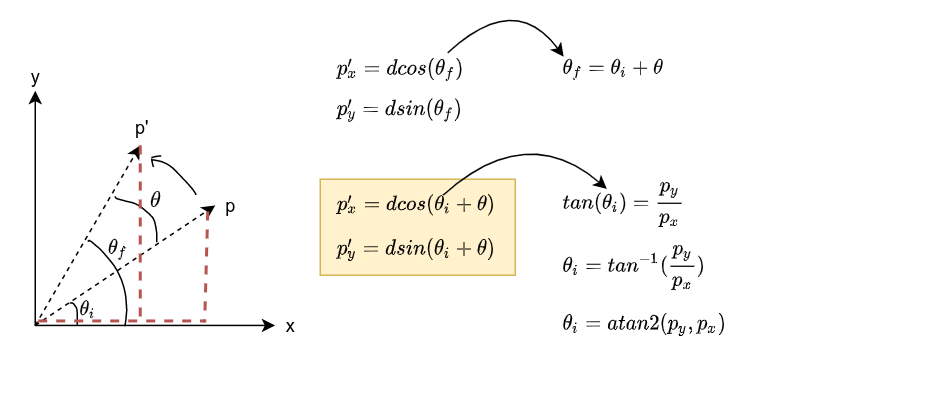You have some point $p$ and you want it to rotate $\theta$ degrees about the origin (0,0).

Most problems, especially geometry oriented math problems, benefit greatly from a drawing, so let’s draw the situation, including what we know ($p$, $\theta$), what we want to know ($p'$), as well as any auxillary elements ($\theta_f$, $\theta_i$, etc) that might helps us figure out a relationship between our known and unknown.

Once we draw the situation, we try to relate the knowns with the unknowns and solve for the unknowns.Here is an implementation of the above solution in iodide.